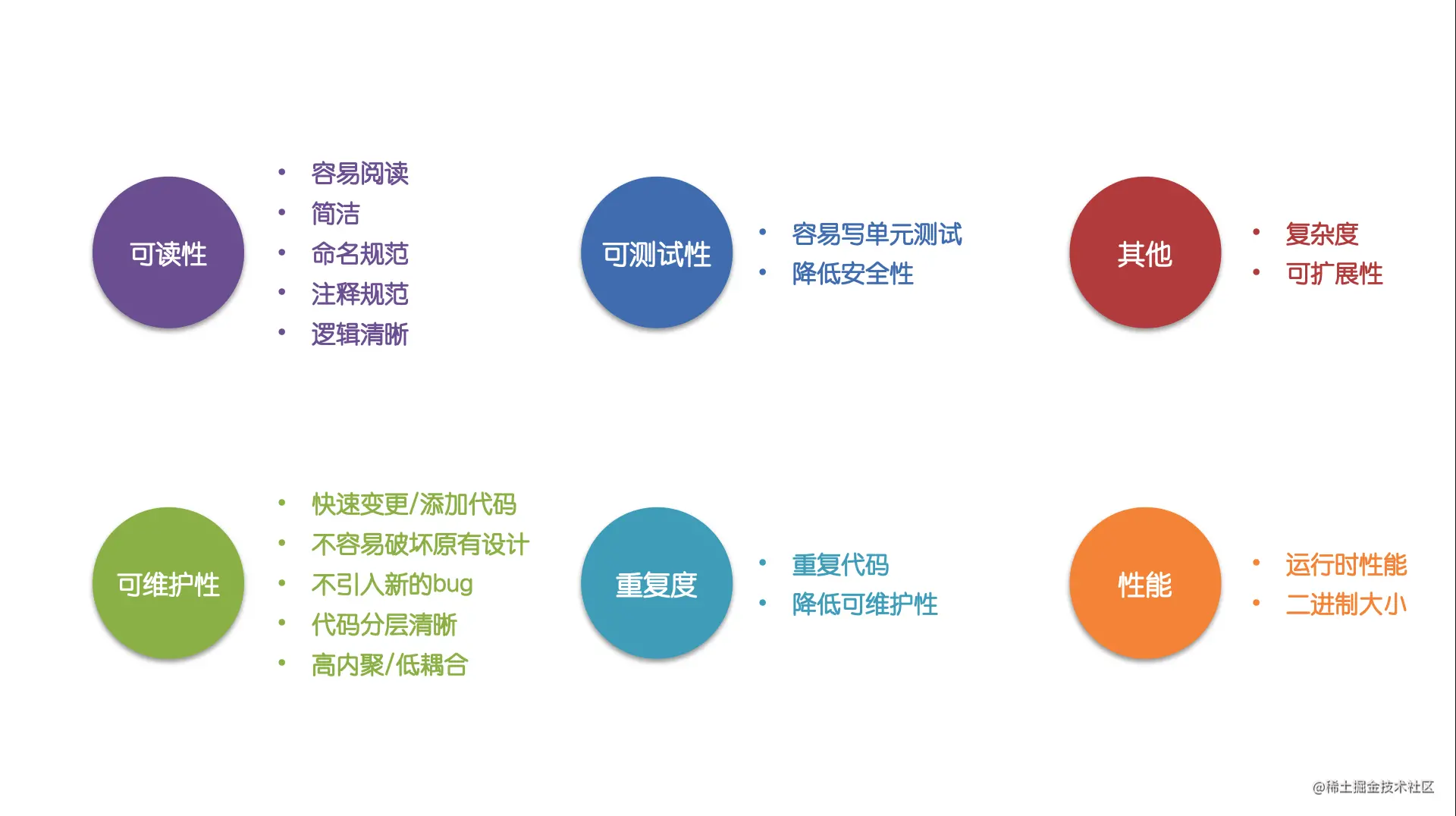Swift 代码质量指标一些不错的实践

1. 利用编译检查

减少使用Any/AnyObject

• 编译器无法检查类型是否正确保证类型安全
• 代码中大量的as?转换
• 类型的缺失导致编译器无法做一些潜在的编译优化
使用as?带来的问题

func do(data: Any?) {
guard let string = data as? String else {
return
}
//
}

do(1)
do("")

使用自定义类型代替Dictionary

• key需要字符串硬编码，编译时无法检查
• value没有类型限制。修改时类型无法限制，读取时需要重复类型转换和解包操作
• 无法利用空安全特性，指定某个属性必须有值

不推荐
let dic: [String: Any]
let num = dic["value"] as? Int
dic["name"] = "name"

推荐
struct Data {
let num: Int
var name: String?
}
let num = data.num
data.name = "name"

适合使用Dictionary的场景
• 数据不使用 - 数据并不读取只是用来传递。
• 解耦
• 1.组件间通信解耦使用HashMap传递参数进行通信。
• 2.跨技术栈边界的场景，混合栈间通信/前后端通信使用HashMap/JSON进行通信。

使用枚举关联值代替Any

优化前
let string = NSMutableAttributedString()

改造后
enum NSAttributedStringKey {
case foregroundColor(UIColor)
}
let string = NSMutableAttributedString()

_

使用KeyPath代替字符串硬编码

KeyPath包含属性名和类型信息，可以避免硬编码字符串，同时当属性名或类型改变时编译器会进行检查。

不推荐
class SomeClass: NSObject {
@objc dynamic var someProperty: Int
init(someProperty: Int) {
self.someProperty = someProperty
}
}
let object = SomeClass(someProperty: 10)
object.observeValue(forKeyPath: "", of: nil, change: nil, context: nil)

推荐
let object = SomeClass(someProperty: 10)
object.observe(.someProperty) { object, change in
}

2. 内存安全

!属性会在读取时隐式强解包，当值不存在时产生运行时异常导致Crash。

class ViewController: UIViewController {
@IBOutlet private var label: UILabel! // @IBOutlet需要使用!
}

减少使用!进行强解包

var num: Int?
let num2 = num! // 错误

try! method()

使用weak/unowned避免循环引用 √

resource.request().onComplete { [weak self] response in
guard let self = self else {
return
}
let model = self.updateModel(response)
self.updateUI(model)
}

resource.request().onComplete { [unowned self] response in
let model = self.updateModel(response)
self.updateUI(model)
}

减少使用unowned

unowned在值不存在时会产生运行时异常导致Crash，只有在确定self一定会存在时才使用unowned

class Class {
@objc unowned var object: Object
@objc weak var object: Object?
}

unowned/weak区别：

• weak - 必须设置为可选值，会进行弱引用处理性能更差。会自动设置为nil
• unowned - 可以不设置为可选值，不会进行弱引用处理性能更好。但是不会自动设置为nil, 如果self已释放会触发错误.

错误处理方式

• 可选值 - 调用方并不关注内部可能会发生错误，当发生错误时返回nil
• try/catch - 明确提示调用方需要处理异常，需要实现Error协议定义明确的错误类型
• assert - 断言。只能在Debug模式下生效
• precondition - 和assert类似，可以再Debug/Release模式下生效
• fatalError - 产生运行时崩溃会导致Crash，应避免使用
• Result - 通常用于闭包异步回调返回值

减少使用可选值

使用init注入代替可选值属性

不推荐
class Object {
var num: Int?
}
let object = Object()
object.num = 1

推荐
class Object {
let num: Int

init(num: Int) {
self.num = num
}
}
let object = Object(num: 1)

避免随意给予可选值默认值

不推荐
func confirmOrder(id: String) {}
// 给予错误的值会导致错误的值被传递到更多的业务流程
confirmOrder(id: orderId ?? "")

推荐
func confirmOrder(id: String) {}

guard let orderId = orderId else {
// 异常处理
return
}
confirmOrder(id: orderId)

使用枚举优化可选值

优化前
class Object {
var name: Int?
var num: Int?
}

优化后
• 降低内存占用 - 枚举关联类型的大小取决于最大的关联类型大小
• 逻辑更清晰 - 使用enum相比大量使用if/else逻辑更清晰
enum CustomType {
case name(String)
case num(Int)
}

减少var属性

使用计算属性

不推荐
class model {
var data: Object?
}
model.data = Object()

推荐
class model {
var data: Object?
return data != nil
}
}
model.data = Object()

使用filter/reduce/map代替for循环

不推荐
let nums = [1, 2, 3]
var result = []
for num in nums {
if num < 3 {
result.append(String(num))
}
}
// result = ["1", "2"]

推荐
let nums = [1, 2, 3]
let result = nums.filter { \$0 < 3 }.map { String(\$0) }
// result = ["1", "2"]

guard !a else {
return
}
guard !b else {
return
}
// do

if a {
if b {
// do
}
}

使用三元运算符?:

推荐
let b = true
let a = b ? 1 : 2

let c: Int?
let b = c ?? 1

var a: Int?
if b {
a = 1
} else {
a = 2
}

使用for where优化循环

for循环添加where语句，只有当where条件满足时才会进入循环

不推荐
for item in collection {
if item.hasProperty {
// ...
}
}

推荐
for item in collection where item.hasProperty {
// item.hasProperty == true，才会进入循环
}

使用defer

defer可以保证在函数退出前一定会执行。可以使用defer中实现退出时一定会执行的操作例如资源释放等避免遗漏。

func method() {
lock.lock()
defer {
lock.unlock()
// 会在method作用域结束的时候调用
}
// do
}

字符串

使用"""

let quotation = """
The White Rabbit put on his spectacles.  "Where shall I begin,

"Begin at the beginning," the King said gravely, "and go on
till you come to the end; then stop."
"""

使用字符串插值

不推荐
let multiplier = 3
let message = String(multiplier) + "times 2.5 is" +
String((Double(multiplier) * 2.5))

推荐
let multiplier = 3
let message = "(multiplier) times 2.5 is (Double(multiplier) * 2.5)"

var nums = []
nums.count == 0
nums

var nums = []
nums.isEmpty
nums.first

访问控制

Swift中默认访问控制级别为internal。编码中应当尽可能减小属性/方法/类型的访问控制级别隐藏内部实现。

使用private/fileprivate修饰私有属性和方法

private let num = 1
class MyClass {
private var num: Int
}

使用private(set)修饰外部只读/内部可读写属性

class MyClass {
private(set) var num = 1
}
let num = MyClass().num
MyClass().num = 2 // 会编译报错

函数

使用参数默认值

不推荐
func test(a: Int, b: String?, c: Int?) {
}
test(1, nil, nil)

推荐
func test(a: Int, b: String? = nil, c: Int? = nil) {
}
test(1)

限制参数数量

不推荐
func f(a: Int, b: Int, c: Int, d: Int, e: Int, f: Int) {
}

推荐
struct Params {
let a, b, c, d, e, f: Int
}
func f(params: Params) {
}

// 上报方法使用方不关心是否成功
func report(id: String) -> Bool {}

@discardableResult func report2(id: String) -> Bool {}

report("1") // 编译器会警告
report2("1") // 不处理返回值编译器不会警告

元组

避免过长的元组

func test() -> (Int, Int, Int) {

}
let (a, b, c) = test()
// a，b，c类型一致，没有命名信息不清楚每个变量的含义
print("a \(a), b: \(b), c: \(c) ")

系统库

block API的优势：

• KVO 可以支持 KeyPath
• 不需要主动移除监听，observer释放时自动移除监听
不推荐
class Object: NSObject {
init() {
super.init()
addObserver(self, forKeyPath: "value", options: .new, context: nil)
}

override class func observeValue(forKeyPath keyPath: String?, of object: Any?, change: [NSKeyValueChangeKey : Any]?, context: UnsafeMutableRawPointer?) {
}

@objc private func test() {
}

deinit {
removeObserver(self, forKeyPath: "value")
}
}

推荐
class Object: NSObject {

private var observer: AnyObserver?
private var kvoObserver: NSKeyValueObservation?

init() {
super.init()
}
kvoObserver = foo.observe(.value, options: [.new]) { (foo, change) in
}
}
}

Protocol

使用protocol代替继承

Swift中针对protocol提供了很多新特性，例如默认实现关联类型，支持值类型。在代码设计时可以优先考虑使用protocol来避免臃肿的父类同时更多使用值类型。

• 1.需要继承NSObject子类。
• 2.需要调用super方法。
• 3.实现抽象类的能力。

Extension

使用extension组织代码

class MyViewController: UIViewController {
// class stuff here
}
// MARK: - Private
extension: MyViewController {
private func method() {}
}
// MARK: - UITableViewDataSource
extension MyViewController: UITableViewDataSource {
// table view data source methods
}
// MARK: - UIScrollViewDelegate
extension MyViewController: UIScrollViewDelegate {
// scroll view delegate methods
}

代码风格

常见的自动格式化修正
• 移除多余的;
• 最多只保留一行换行
• 自动对齐空格
• 限制每行的宽度自动换行

Swift 编程官网规范

————————————————————————————————————————————